You are currently offline. Some features of the site may not work correctly.

# Computable isomorphism

Known as: Computably isomorphic
In computability theory two sets of natural numbers are computably isomorphic or recursively isomorphic if there exists a total bijective computable… Expand
Wikipedia

## Papers overview

Semantic Scholar uses AI to extract papers important to this topic.
2019
2019
• Arch. Math. Log.
• 2019
• Corpus ID: 85562879
A major theme in the study of degree structures of all types has been the question of the decidability or undecidability of their… Expand
Is this relevant?
2015
2015
We show the equivalence between the existence of winning strategies for $G_{\delta \sigma}$ (also called $\Sigma^{0}_{3}$) games… Expand
•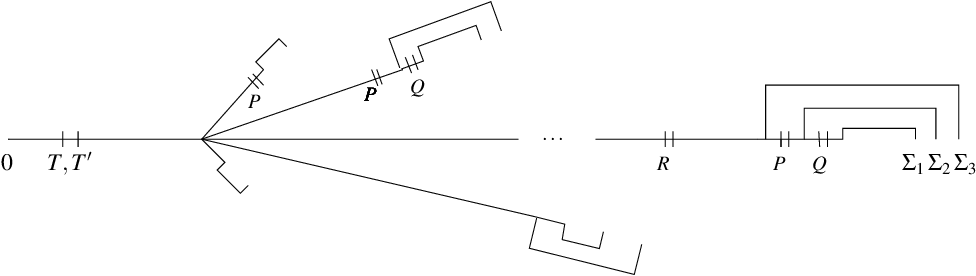Is this relevant?
2005
2005
• Discret. Appl. Math.
• 2005
• Corpus ID: 3819753
In this paper, we examine the hard problems underlying asymmetric pairings, their precise relationships and how they affect a… Expand
•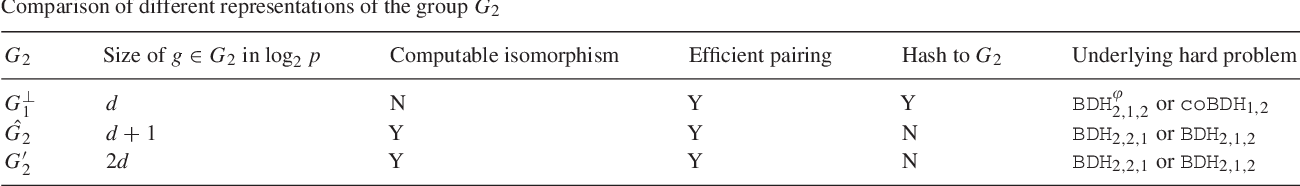Is this relevant?
2004
2004
Quantified propositional intuitionistic logic is obtained from propositional intuitionistic logic by adding quantifiers ∀p, ∃p… Expand
Is this relevant?
1998
1998
• Ann. Pure Appl. Log.
• 1998
• Corpus ID: 1484207
Abstract The spectrum of a relation R on a computable structure is the set of Turing degrees of the image of R under all… Expand
Is this relevant?
1998
1998
• Ann. Pure Appl. Log.
• 1998
• Corpus ID: 391207
Abstract A computable graph is constructed which is not computably isomorphic to any polynomial-time graph with a standard… Expand
Is this relevant?
1997
1997
We define a propositionally quantified intuitionistic logic Her+ by a natural extension of Kripke's semantics for propositional… Expand
•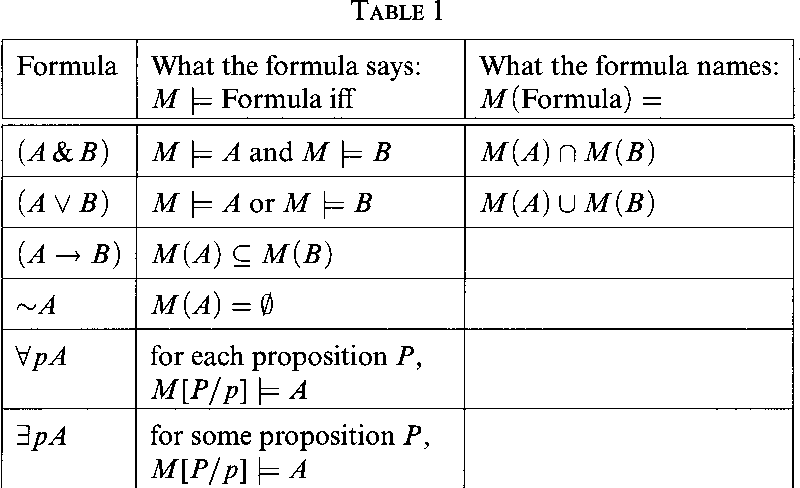•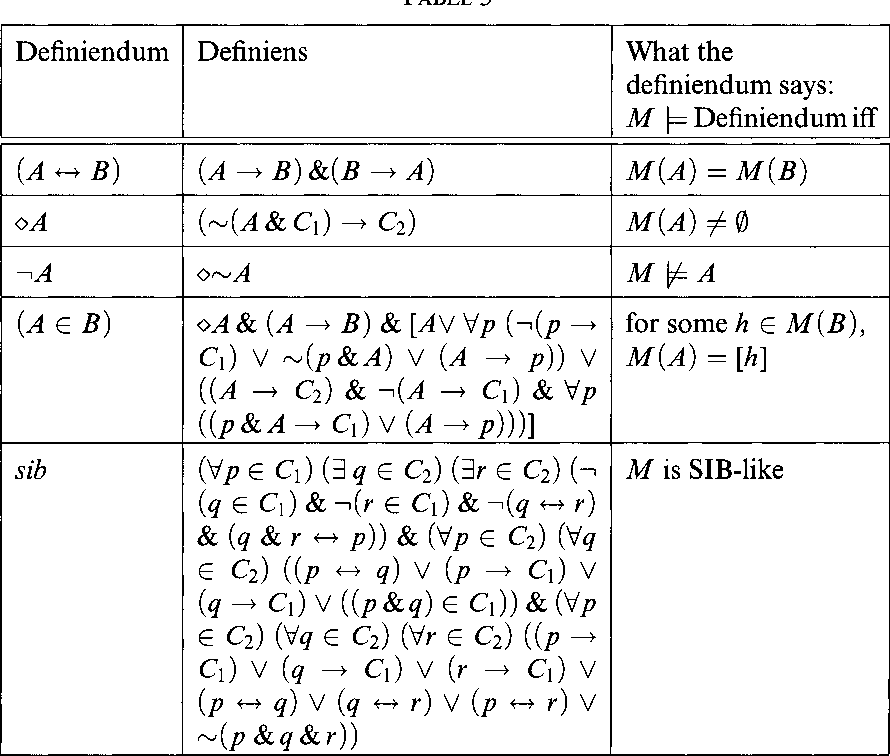•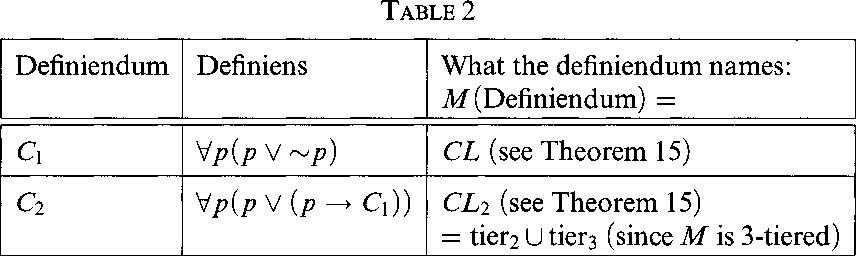•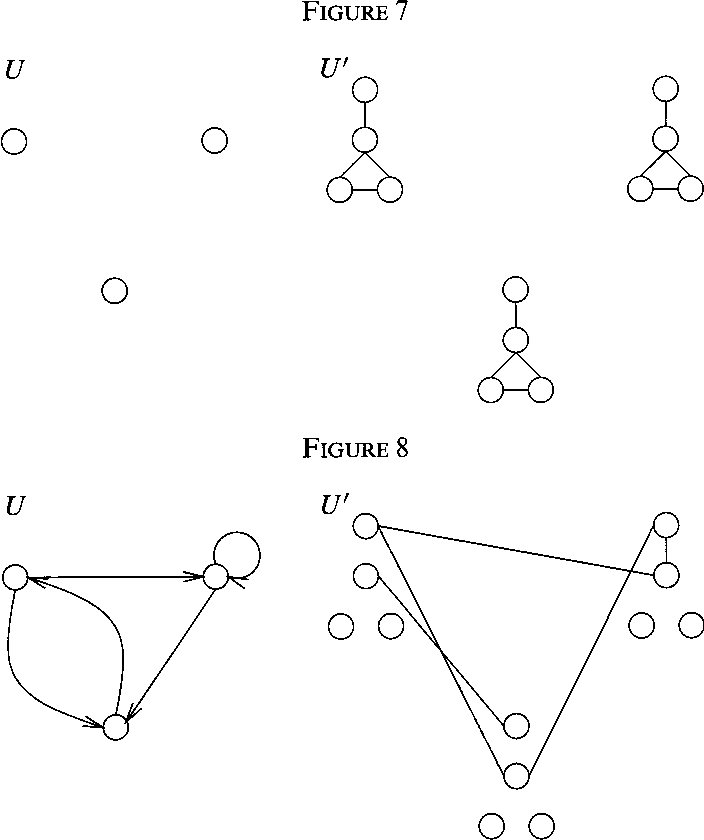Is this relevant?
1997
1997
• Arch. Math. Log.
• 1997
• Corpus ID: 9984550
Abstract. We prove that every countable relation on the enumeration degrees, ${\frak E}$, is uniformly definable from parameters… Expand
Is this relevant?
1989
1989
Culik and Yu suggested a classification of cellular a u­ tomata into four classes based on Wolfram's earlier heuristic classifi… Expand
Is this relevant?
1985
1985
• Theor. Comput. Sci.
• 1985
• Corpus ID: 9075800
Abstract A set A is polynomial many-one reducible to a set B ( A is Karp-reducible to B ) if there is a polynomially computable… Expand
Is this relevant?